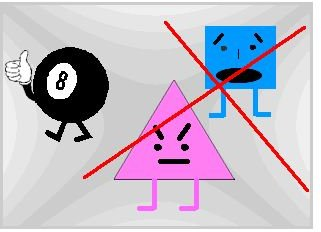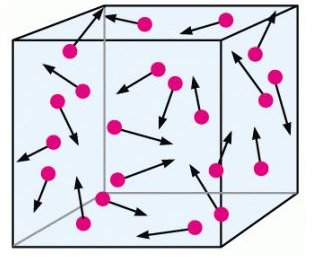# The Postulates of the Kinetic Theory of Gases Part One

## Introduction

The kinetic theory of gases is based on a microscopic molecular model. Here, large scale objects, in our case an ideal gas, can be treated as a collection of molecules. This model is essential for subsequent applications in areas such as statistical physics, also known as statistical mechanics or statistical thermodynamics. But underlying this successful theory are several important assumptions that are based on Newtonian mechanics. Understanding these assumptions makes it easier to get a handle on such topics as the molecular explanation of temperature. These assumptions also explain the relationship between temperature, pressure, and volume in gases.

Before proceeding, you may wish to review the Bright Hub article on energy for a refresher on the definitions of kinetic and potential energy. Simply put, kinetic energy is the energy of motion while potential energy is stored energy.

You will also need to recall from basic physics the concept of momentum, which is an inertia of motion (momentum = mass x velocity).

Without further ado, let us proceed.

## The Number of Molecules

There is a large number of molecules N, each of which has a mass m. This actually makes sense in light of our previous explorations with ideal gases; in a previous article we learned how to calculate the number of air molecules in a room, and found this to be a tremendously large number.

## Shape of the Molecules

The moleculesare assumed to be spherical in shape, like billiards or soccer balls or baseballs.

## Gas Type

The gases are pure, so the molecules are assumed to be identical and we are unable to distinguish between them. This also makes it easier to count them.

## Molecular Separation

The average separationof the molecules is large compared with their dimensions. This means that if the molecules have a diameter d, and they are separated by a distance R, then R >> d (R is much greater than d). In other words, the molecules are tiny and must be far apart.

This also means that these molecules are tiny enough so they can be considered point masses, and so we don’t take into account their internal structure when we use our models or fashion new ones.

## Molecular Motion

As mentioned, the molecules are assumed to obey Newton’s laws of motion. However, this motion is random;the molecules move in all directions at equal probabilities. They also have varying speeds. Even when they can collide with each other, this random motion remains the same, i.e. the distribution of these velocities do not change with time.

What do bouncing billard balls, the unfortunate collision of a scarecrow with an electrical pole, and Boyle’s Law have to do with the kinetic theory of gases? Continue on to part two if you wish to find out.

Image

QuantumFreak.com

## This post is part of the series: Introduction to the Kinetic Theory of Gases

This is a series that explains the underlying assumptions of the kinetic theory of gases and why they are important. Part one explains physical properties of the molecules such as their shape and number. Part two explains how the collisions of molecules within a container lead to Boyle’s Law.